# SAT Math Multiple Choice Question 977: Answer and Explanation

### Test Information

Question: 977

13. The center of circle O (not shown) falls on the point where the line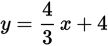intersects the x-axis on the coordinate plane. The point (3, 8) lies on the circumference of the circle.Which of the following could be the equation for circle O?

• A.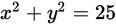• B.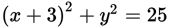• C.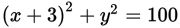• D.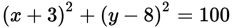Explanation:

C

Difficulty: Hard

Category: Additional Topics in Math / Geometry

Strategic Advice: If you know the center and the radius of a circle, you can write its equation in the form (x - h)2 + (y - k)2 = r2, where (h, k) is the center of the circle and r is the length of its radius.

Getting to the Answer: Start by finding the center of the circle. You're told that the center falls on the point where the given line intersects the x-axis, which is the same as the x-intercept of the line. An x-intercept is always of the form (x, 0), so set y = 0 and solve for x: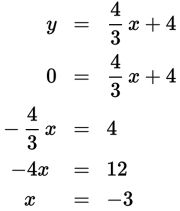You now know that the center of circle O is (-3, 0), so its equation should look like (x - (-3))2 + (y - 0)2 = r2 or, when simplified, (x + 3)2 + y2 = r2. This means you can eliminate A and D.

To find the radius of the circle, draw a quick sketch that includes the center of the circle and the point given in the question, (3, 8).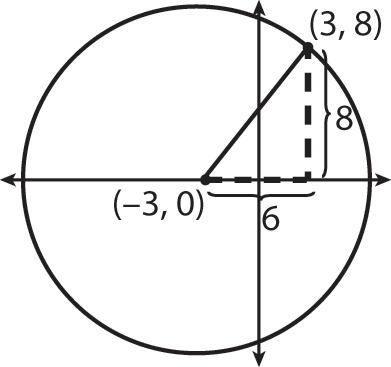Notice that drawing in a right triangle from the center of the circle to the point on the circumference gives you a nice 6-8-10 Pythagorean triplet, making the radius of the circle 10 (or you could use the Pythagorean theorem to find the length of the radius). Thus, the equation of the circle is (x + 3)2 + y2 = 102, or (r + 3)2 + y2 = 100, making (C) correct.